# Spectral analysis#

Perform a full region based on-off spectral analysis and fit the resulting datasets.

## Prerequisites#

• Understanding how spectral extraction is performed in Cherenkov astronomy, in particular regarding OFF background measurements.

• Understanding the basics data reduction and modeling/fitting process with the gammapy library API as shown in the Low level API tutorial.

## Context#

While 3D analyses allow in principle to consider complex field of views containing overlapping gamma-ray sources, in many cases we might have an observation with a single, strong, point-like source in the field of view. A spectral analysis, in that case, might consider all the events inside a source (or ON) region and bin them in energy only, obtaining 1D datasets.

In classical Cherenkov astronomy, the background estimation technique associated with this method measures the number of events in OFF regions taken in regions of the field-of-view devoid of gamma-ray emitters, where the background rate is assumed to be equal to the one in the ON region.

This allows to use a specific fit statistics for ON-OFF measurements, the wstat (see `wstat`), where no background model is assumed. Background is treated as a set of nuisance parameters. This removes some systematic effects connected to the choice or the quality of the background model. But this comes at the expense of larger statistical uncertainties on the fitted model parameters.

Objective: perform a full region based spectral analysis of 4 Crab observations of H.E.S.S. data release 1 and fit the resulting datasets.

## Introduction#

Here, as usual, we use the `DataStore` to retrieve a list of selected observations (`Observations`). Then, we define the ON region containing the source and the geometry of the `SpectrumDataset` object we want to produce. We then create the corresponding dataset Maker.

We have to define the Maker object that will extract the OFF counts from reflected regions in the field-of-view. To ensure we use data in an energy range where the quality of the IRFs is good enough we also create a safe range Maker.

We can then proceed with data reduction with a loop over all selected observations to produce datasets in the relevant geometry.

We can then explore the resulting datasets and look at the cumulative signal and significance of our source. We finally proceed with model fitting.

In practice, we have to:

```from pathlib import Path

# Check package versions
import numpy as np
import astropy.units as u
from astropy.coordinates import Angle, SkyCoord
from regions import CircleSkyRegion

# %matplotlib inline
import matplotlib.pyplot as plt
```

## Setup#

```from IPython.display import display
from gammapy.data import DataStore
from gammapy.datasets import (
Datasets,
FluxPointsDataset,
SpectrumDataset,
SpectrumDatasetOnOff,
)
from gammapy.estimators import FluxPointsEstimator
from gammapy.makers import (
ReflectedRegionsBackgroundMaker,
SpectrumDatasetMaker,
)
from gammapy.maps import MapAxis, RegionGeom, WcsGeom
from gammapy.modeling import Fit
from gammapy.modeling.models import (
ExpCutoffPowerLawSpectralModel,
SkyModel,
create_crab_spectral_model,
)
```

## Check setup#

```from gammapy.utils.check import check_tutorials_setup
from gammapy.visualization import plot_spectrum_datasets_off_regions

check_tutorials_setup()
```
```System:

python_executable      : /home/runner/work/gammapy-docs/gammapy-docs/gammapy/.tox/build_docs/bin/python
python_version         : 3.9.16
machine                : x86_64
system                 : Linux

Gammapy package:

version                : 1.1
path                   : /home/runner/work/gammapy-docs/gammapy-docs/gammapy/.tox/build_docs/lib/python3.9/site-packages/gammapy

Other packages:

numpy                  : 1.24.3
scipy                  : 1.10.1
astropy                : 5.2.2
regions                : 0.7
click                  : 8.1.3
yaml                   : 6.0
IPython                : 8.14.0
jupyterlab             : not installed
matplotlib             : 3.7.1
pandas                 : not installed
healpy                 : 1.16.2
iminuit                : 2.21.3
sherpa                 : 4.15.1
naima                  : 0.10.0
emcee                  : 3.1.4
corner                 : 2.2.2
ray                    : 2.5.0

Gammapy environment variables:

GAMMAPY_DATA           : /home/runner/work/gammapy-docs/gammapy-docs/gammapy-datasets/1.1
```

First, we select and load some H.E.S.S. observations of the Crab nebula (simulated events for now).

We will access the events, effective area, energy dispersion, livetime and PSF for containment correction.

```datastore = DataStore.from_dir("\$GAMMAPY_DATA/hess-dl3-dr1/")
obs_ids = [23523, 23526, 23559, 23592]
observations = datastore.get_observations(obs_ids)
```

## Define Target Region#

The next step is to define a signal extraction region, also known as on region. In the simplest case this is just a CircleSkyRegion.

```target_position = SkyCoord(ra=83.63, dec=22.01, unit="deg", frame="icrs")
```

We will use the reflected regions method to place off regions to estimate the background level in the on region. To make sure the off regions don’t contain gamma-ray emission, we create an exclusion mask.

Using http://gamma-sky.net/ we find that there’s only one known gamma-ray source near the Crab nebula: the AGN called RGB J0521+212 at GLON = 183.604 deg and GLAT = -8.708 deg.

```exclusion_region = CircleSkyRegion(
center=SkyCoord(183.604, -8.708, unit="deg", frame="galactic"),
)

skydir = target_position.galactic
geom = WcsGeom.create(
npix=(150, 150), binsz=0.05, skydir=skydir, proj="TAN", frame="icrs"
)

plt.show()
```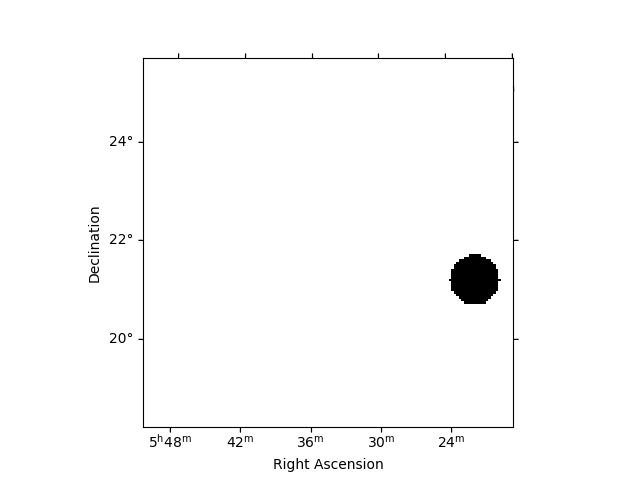## Run data reduction chain#

We begin with the configuration of the maker classes:

```energy_axis = MapAxis.from_energy_bounds(
0.1, 40, nbin=10, per_decade=True, unit="TeV", name="energy"
)
energy_axis_true = MapAxis.from_energy_bounds(
0.05, 100, nbin=20, per_decade=True, unit="TeV", name="energy_true"
)

geom = RegionGeom.create(region=on_region, axes=[energy_axis])
dataset_empty = SpectrumDataset.create(geom=geom, energy_axis_true=energy_axis_true)

dataset_maker = SpectrumDatasetMaker(
containment_correction=True, selection=["counts", "exposure", "edisp"]
)
```
```datasets = Datasets()

for obs_id, observation in zip(obs_ids, observations):
dataset = dataset_maker.run(dataset_empty.copy(name=str(obs_id)), observation)
dataset_on_off = bkg_maker.run(dataset, observation)
datasets.append(dataset_on_off)

print(datasets)
```
```Datasets
--------

Dataset 0:

Type       : SpectrumDatasetOnOff
Name       : 23523
Instrument : HESS
Models     :

Dataset 1:

Type       : SpectrumDatasetOnOff
Name       : 23526
Instrument : HESS
Models     :

Dataset 2:

Type       : SpectrumDatasetOnOff
Name       : 23559
Instrument : HESS
Models     :

Dataset 3:

Type       : SpectrumDatasetOnOff
Name       : 23592
Instrument : HESS
Models     :
```

## Plot off regions#

```plt.figure()
on_region.to_pixel(ax.wcs).plot(ax=ax, edgecolor="k")
plot_spectrum_datasets_off_regions(ax=ax, datasets=datasets)
plt.show()
```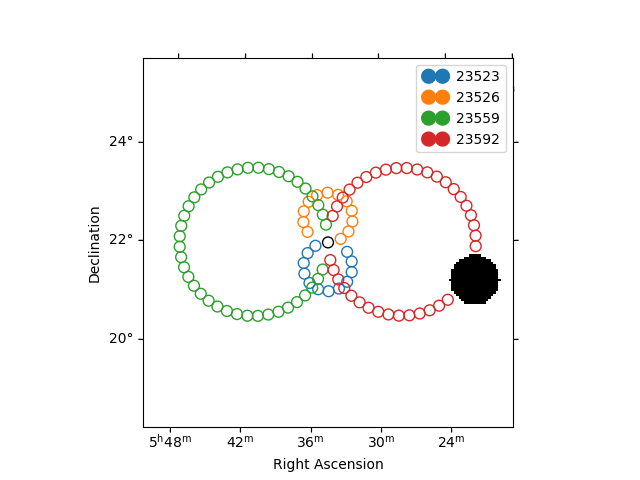```/home/runner/work/gammapy-docs/gammapy-docs/gammapy/.tox/build_docs/lib/python3.9/site-packages/regions/shapes/circle.py:161: UserWarning: Setting the 'color' property will override the edgecolor or facecolor properties.
/home/runner/work/gammapy-docs/gammapy-docs/gammapy/.tox/build_docs/lib/python3.9/site-packages/gammapy/visualization/datasets.py:84: UserWarning: Setting the 'color' property will override the edgecolor or facecolor properties.
handle = Patch(**plot_kwargs)
```

## Source statistic#

Next we’re going to look at the overall source statistics in our signal region.

```info_table = datasets.info_table(cumulative=True)

display(info_table)
```
```  name  counts       excess      ...   acceptance_off          alpha
...
------- ------ ----------------- ... ------------------ -------------------
stacked    149            139.25 ...              216.0  0.0833333358168602
stacked    303            280.75 ... 227.99996948242188  0.0833333432674408
stacked    439 408.7743835449219 ...  373.3919677734375 0.05088486522436142
stacked    550  512.135498046875 ... 436.05487060546875 0.04357249662280083
```

And make the corresponding plots

```fig, (ax_excess, ax_sqrt_ts) = plt.subplots(figsize=(10, 4), ncols=2, nrows=1)
ax_excess.plot(
info_table["livetime"].to("h"),
info_table["excess"],
marker="o",
ls="none",
)

ax_excess.set_title("Excess")
ax_excess.set_xlabel("Livetime [h]")
ax_excess.set_ylabel("Excess events")

ax_sqrt_ts.plot(
info_table["livetime"].to("h"),
info_table["sqrt_ts"],
marker="o",
ls="none",
)

ax_sqrt_ts.set_title("Sqrt(TS)")
ax_sqrt_ts.set_xlabel("Livetime [h]")
ax_sqrt_ts.set_ylabel("Sqrt(TS)")
plt.show()
```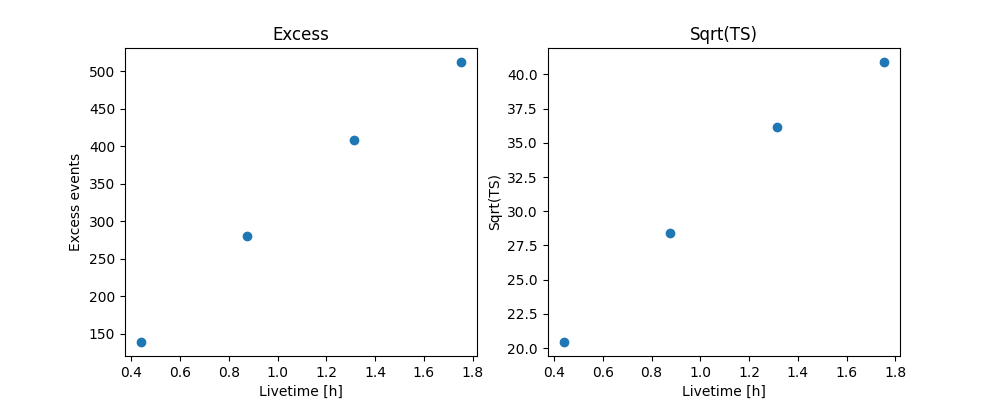Finally you can write the extracted datasets to disk using the OGIP format (PHA, ARF, RMF, BKG, see here for details):

```path = Path("spectrum_analysis")
path.mkdir(exist_ok=True)

for dataset in datasets:
dataset.write(filename=path / f"obs_{dataset.name}.fits.gz", overwrite=True)
```

If you want to read back the datasets from disk you can use:

```datasets = Datasets()

for obs_id in obs_ids:
filename = path / f"obs_{obs_id}.fits.gz"
```

## Fit spectrum#

Now we’ll fit a global model to the spectrum. First we do a joint likelihood fit to all observations. If you want to stack the observations see below. We will also produce a debug plot in order to show how the global fit matches one of the individual observations.

```spectral_model = ExpCutoffPowerLawSpectralModel(
amplitude=1e-12 * u.Unit("cm-2 s-1 TeV-1"),
index=2,
lambda_=0.1 * u.Unit("TeV-1"),
reference=1 * u.TeV,
)
model = SkyModel(spectral_model=spectral_model, name="crab")

datasets.models = [model]

fit_joint = Fit()
result_joint = fit_joint.run(datasets=datasets)

# we make a copy here to compare it later
model_best_joint = model.copy()
```

## Fit quality and model residuals#

We can access the results dictionary to see if the fit converged:

```print(result_joint)
```
```OptimizeResult

backend    : minuit
success    : True
message    : Optimization terminated successfully..
nfev       : 244
total stat : 86.12

CovarianceResult

backend    : minuit
method     : hesse
success    : True
message    : Hesse terminated successfully.
```

and check the best-fit parameters

```display(result_joint.models.to_parameters_table())
```
```model   type      name     value         unit      ... max frozen is_norm link
----- -------- --------- ---------- -------------- ... --- ------ ------- ----
crab spectral     index 2.2727e+00                ... nan  False   False
crab spectral amplitude 4.7913e-11 cm-2 s-1 TeV-1 ... nan  False    True
crab spectral reference 1.0000e+00            TeV ... nan   True   False
crab spectral   lambda_ 1.2097e-01          TeV-1 ... nan  False   False
crab spectral     alpha 1.0000e+00                ... nan   True   False
```

A simple way to inspect the model residuals is using the function `plot_fit()`

```ax_spectrum, ax_residuals = datasets.plot_fit()
ax_spectrum.set_ylim(0.1, 40)
plt.show()
```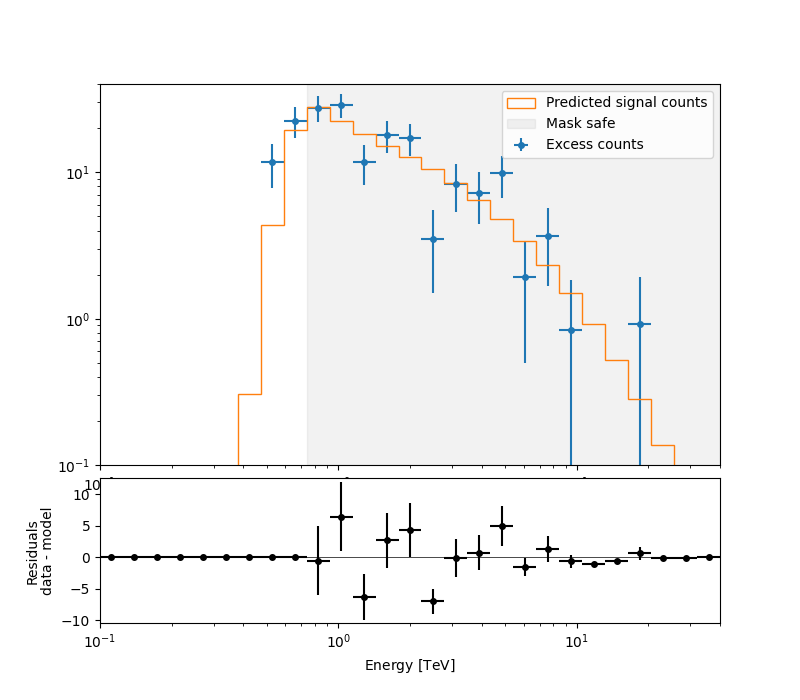For more ways of assessing fit quality, please refer to the dedicated Fitting tutorial.

## Compute Flux Points#

To round up our analysis we can compute flux points by fitting the norm of the global model in energy bands. We’ll use a fixed energy binning for now:

```e_min, e_max = 0.7, 30
energy_edges = np.geomspace(e_min, e_max, 11) * u.TeV
```

Now we create an instance of the `FluxPointsEstimator`, by passing the dataset and the energy binning:

```fpe = FluxPointsEstimator(
energy_edges=energy_edges, source="crab", selection_optional="all"
)
flux_points = fpe.run(datasets=datasets)
```

Here is a the table of the resulting flux points:

```display(flux_points.to_table(sed_type="dnde", formatted=True))
```
```e_ref  e_min  e_max  ... success   norm_scan        stat_scan
TeV    TeV    TeV   ...
------ ------ ------ ... ------- -------------- ------------------
0.823  0.737  0.920 ...    True 0.200 .. 5.000 140.739 .. 451.124
1.148  0.920  1.434 ...    True 0.200 .. 5.000 182.603 .. 693.653
1.790  1.434  2.235 ...    True 0.200 .. 5.000 150.799 .. 424.855
2.790  2.235  3.483 ...    True 0.200 .. 5.000 102.546 .. 293.446
3.892  3.483  4.348 ...    True 0.200 .. 5.000  13.594 .. 125.092
5.429  4.348  6.778 ...    True 0.200 .. 5.000  47.101 .. 132.109
8.462  6.778 10.564 ...    True 0.200 .. 5.000   28.474 .. 55.783
11.803 10.564 13.189 ...    True 0.200 .. 5.000   12.285 .. 18.679
16.465 13.189 20.556 ...    True 0.200 .. 5.000    9.622 .. 17.557
25.663 20.556 32.040 ...    True 0.200 .. 5.000     0.866 .. 6.774
```

Now we plot the flux points and their likelihood profiles. For the plotting of upper limits we choose a threshold of TS < 4.

```fig, ax = plt.subplots()
flux_points.plot(ax=ax, sed_type="e2dnde", color="darkorange")
flux_points.plot_ts_profiles(ax=ax, sed_type="e2dnde")
plt.show()
```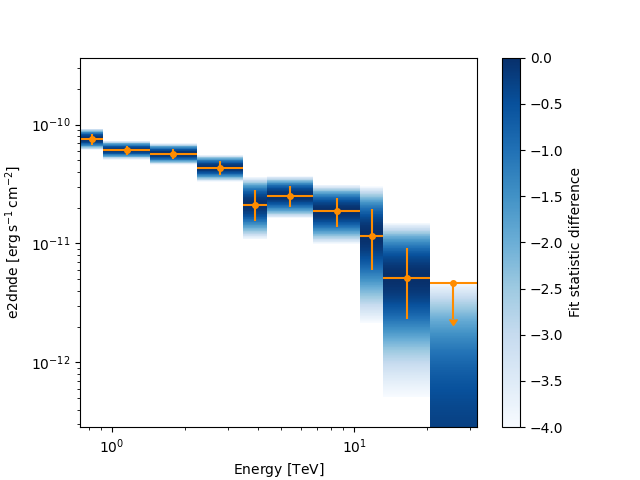The final plot with the best fit model, flux points and residuals can be quickly made like this:

```flux_points_dataset = FluxPointsDataset(data=flux_points, models=model_best_joint)
flux_points_dataset.plot_fit()
plt.show()
```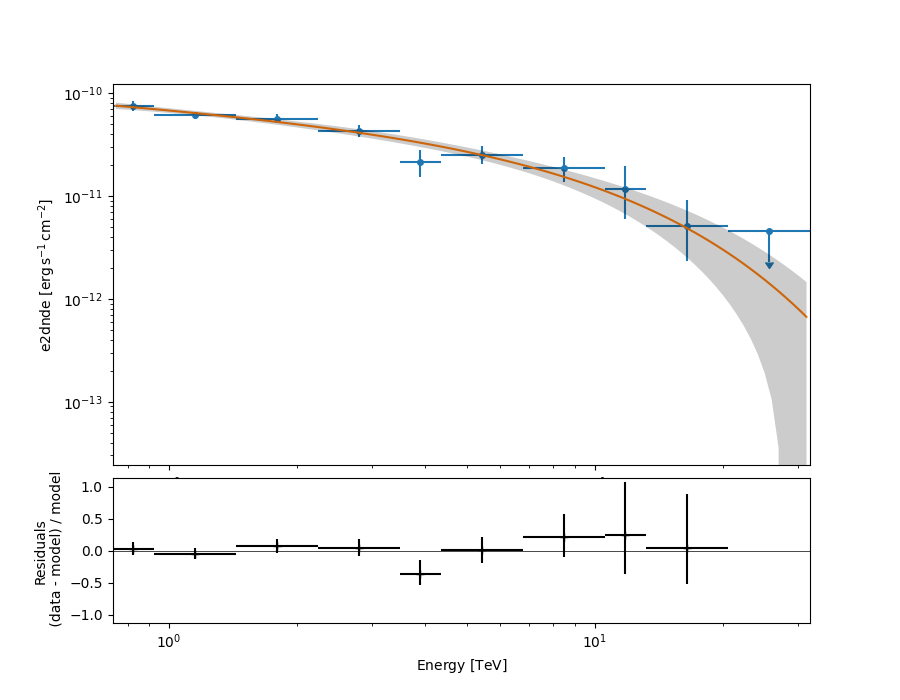## Stack observations#

An alternative approach to fitting the spectrum is stacking all observations first and the fitting a model. For this we first stack the individual datasets:

```dataset_stacked = Datasets(datasets).stack_reduce()
```

Again we set the model on the dataset we would like to fit (in this case it’s only a single one) and pass it to the `Fit` object:

```dataset_stacked.models = model
stacked_fit = Fit()
result_stacked = stacked_fit.run([dataset_stacked])

# make a copy to compare later
model_best_stacked = model.copy()

print(result_stacked)
```
```OptimizeResult

backend    : minuit
success    : True
message    : Optimization terminated successfully..
nfev       : 54
total stat : 8.16

CovarianceResult

backend    : minuit
method     : hesse
success    : True
message    : Hesse terminated successfully.
```

And display the parameter table

```display(model_best_joint.parameters.to_table())

display(model_best_stacked.parameters.to_table())
```
```  type      name     value         unit      ... max frozen is_norm link
-------- --------- ---------- -------------- ... --- ------ ------- ----
spectral     index 2.2727e+00                ... nan  False   False
spectral amplitude 4.7913e-11 cm-2 s-1 TeV-1 ... nan  False    True
spectral reference 1.0000e+00            TeV ... nan   True   False
spectral   lambda_ 1.2097e-01          TeV-1 ... nan  False   False
spectral     alpha 1.0000e+00                ... nan   True   False
type      name     value         unit      ... max frozen is_norm link
-------- --------- ---------- -------------- ... --- ------ ------- ----
spectral     index 2.2785e+00                ... nan  False   False
spectral amplitude 4.7800e-11 cm-2 s-1 TeV-1 ... nan  False    True
spectral reference 1.0000e+00            TeV ... nan   True   False
spectral   lambda_ 1.1830e-01          TeV-1 ... nan  False   False
spectral     alpha 1.0000e+00                ... nan   True   False
```

Finally, we compare the results of our stacked analysis to a previously published Crab Nebula Spectrum for reference. This is available in `create_crab_spectral_model`.

```fig, ax = plt.subplots()

plot_kwargs = {
"energy_bounds": [0.1, 30] * u.TeV,
"sed_type": "e2dnde",
"yunits": u.Unit("erg cm-2 s-1"),
"ax": ax,
}

# plot stacked model
model_best_stacked.spectral_model.plot(**plot_kwargs, label="Stacked analysis result")
model_best_stacked.spectral_model.plot_error(facecolor="blue", alpha=0.3, **plot_kwargs)

# plot joint model
model_best_joint.spectral_model.plot(
**plot_kwargs, label="Joint analysis result", ls="--"
)
model_best_joint.spectral_model.plot_error(facecolor="orange", alpha=0.3, **plot_kwargs)

create_crab_spectral_model("hess_ecpl").plot(
**plot_kwargs,
label="Crab reference",
)
ax.legend()
plt.show()

# sphinx_gallery_thumbnail_number = 5
```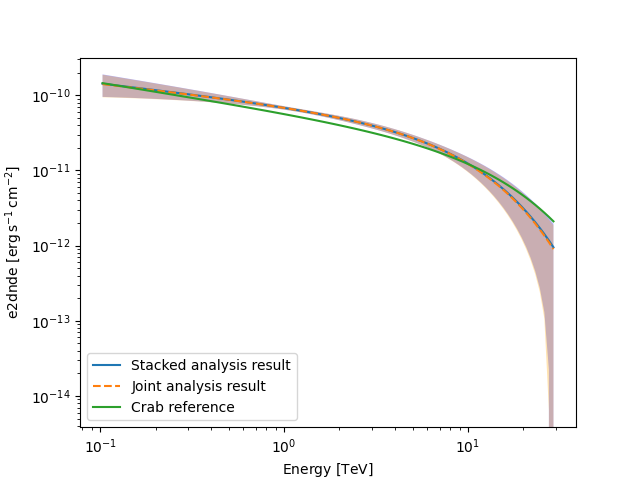## Exercises#

Now you have learned the basics of a spectral analysis with Gammapy. To practice you can continue with the following exercises:

## What next?#

The methods shown in this tutorial is valid for point-like or midly extended sources where we can assume that the IRF taken at the region center is valid over the whole region. If one wants to extract the 1D spectrum of a large source and properly average the response over the extraction region, one has to use a different approach explained in the Spectral analysis of extended sources tutorial.

Total running time of the script: ( 0 minutes 17.667 seconds)

Gallery generated by Sphinx-Gallery